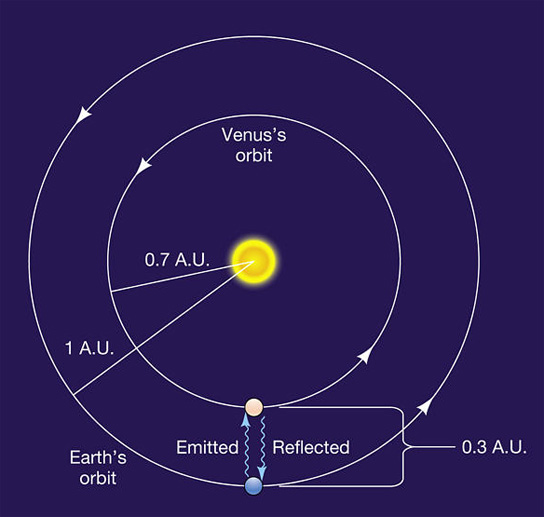# IAU Changes the Astronomical Unit from Equation to NumberAstronomers have redefined one of the most commonly used stellar distances, the astronomical unit (AU). Based on the rough distance from Sol to Earth, AU has been transformed from a confusing equation to simple number.

Astronomers had adopted this simplification at this summer’s past International Astronomical Union’s meeting in Beijing, China, making the astronomical unit (AU) 149,597,870,700 meters.Previously, the AU was known as the radius of an unperturbed circular Newtonian orbit about the Sun of a particle having infinitesimal mass, moving with a mean motion of 0.01720209895 radians per day (known as the Gaussian constant).

While it baffled astronomy students, it also clashed with Einstein’s general theory of relativity, which tended to shift the AU’s distance by about a thousand kilometers, depending on where the observer is located. Traditionally, the AU is linked to the mass of Sol, but since Sol is continually losing mass, the AU has been slowly changing as well.

With the AU being a single number, it simplifies all of these problems and stays away from issues linked to the location of the observer. It is now a fixed number of kilometers, which is far easier to understand.

[via Nature]

#### 8 Commentson "IAU Changes the Astronomical Unit from Equation to Number"

1.Denis | September 14, 2012 at 9:42 am | Reply

I understand how the old method suffered from relativistic effects, but not sure why you say the new one doesn’t? A meter rod will change lengtn depending on its distance from the Sun and other gravity wells.

2.David | September 14, 2012 at 10:48 am | Reply

149 billion
597 million
870 thousand
700 meters.

3.Jake | September 14, 2012 at 10:53 pm | Reply

I don’t see how an AU can be one single number, since earth’s orbit around the sun is eliptical not circular.

4.Marku Demitrius | September 15, 2012 at 6:26 pm | Reply

I find it noteworthy that nowhere did the article mention an AU was 93 million miles, which is the distance from our sun to the earth.

5.Madanagopal.V.C. | September 16, 2012 at 12:50 pm | Reply

1 A.U is the astronomical unit denoting roughly 149 million kilometers or 93 million miles, being the average distance of Earth to Sun. Physics will never be satisfied with averages. We will probe as exactly as possible just as in the case of speed of light which is calculated correct upto 299 792 458 metres. We don`t keep quiet taking it as 300 million meters. Why? Even in the case of pi value, compputer is keeping on calculating its value for umpty number of digits continously. So, it is correct that astronomers take the value of 1 Astronomical Unit as 149 597 870 700 metres being a precise unit of lenght rather than vague average distance of Earth to Sun which will always vary. Thus the IAU changes are long overdue for the benefit of precise physics calculations, just as they discarded the planet Pluto, demoted to be a Kuiper Belt Object much to the displeasure of common man discarding nine planets theory. Thank You.

6.Ron Stewart | September 18, 2012 at 5:52 am | Reply

I believe the last comment to be correct. However, that would also actually equal 149,597.870700 (km)-(kilometers) to be correct in (km) measurement.

7.pappootty.k | September 22, 2012 at 2:00 am | Reply

Why not fix 1AU as 150 million km and state distance of sun at any time as so many AU, eg.0.998AU etc.

8.phil marcahnd | November 8, 2014 at 5:14 pm | Reply

Well, the AU is not a metric unit, even though it is now defined in meters.
So, the AU is obsolete.
Even astronomers will adapt the metric system and use the meter as a measure of length/distance.
The AU is thus nearly 150 gigameter (gm) or 0.15 tm